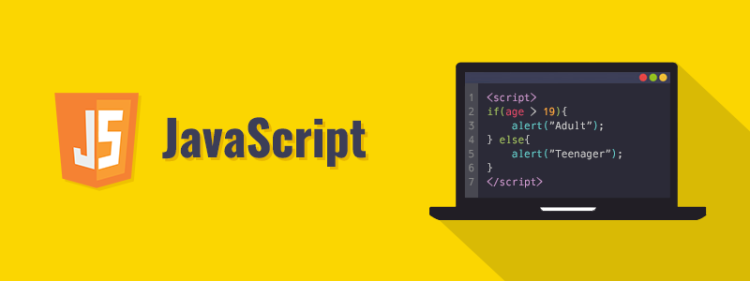← back to the blog

# Project Euler 182 solution JavaScript

##### Posted on July 3rd, 2020 in JavaScript by GeorgeThis is the javascript solution for Project Euler 182.

The final mathematical solution does not belong to me.

I have translated the solution from Java to JavaScript from here https://github.com/kkmonlee/Project-Euler-Solutions/blob/master/Java/p182.java.

I hope this will help others to explore the problem using JavaScript because for some people, the learning process works better through reverse engineering.

``````// *** Define constants ***

const p = 1009;
const q = 3643;

let phi = (p - 1) * (q - 1);

//Helper function GCD of two numbers
// *** Calculate the greatest common divisor ***

const gcd = (x, y) => {
while (y) {
// temp hold y value
var t = y;
// get reminder from x modulus y
y = x % y;
//assign temp to x
x = t;
}
return x;
}

// *** Solution ***

function euler182() {

let min = Infinity;
let noUnconcealedMsgs = 0;

for (var e = 2; e <= phi; e++) {

if (gcd(e, phi) == 1) {

noUnconcealedMsgs = (gcd(e - 1, p - 1) + 1) * (gcd(e - 1, q - 1) + 1);

if (noUnconcealedMsgs < min) {
min = noUnconcealedMsgs;
}

if (noUnconcealedMsgs == min) {